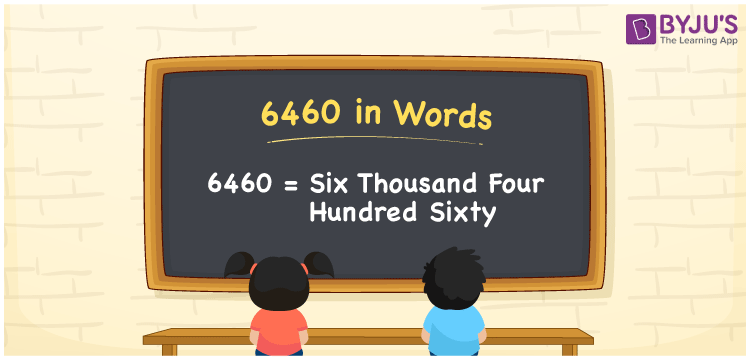# 6460 in Words

6460 in words can be written as Six Thousand Four Hundred Sixty. Suppose if you buy a computer for Rs. 6460, then you can say that “I bought a computer for Six Thousand Four Hundred Sixty Rupees”. For a better hold on the numbers in words concept, students can refer to the reference materials available at BYJU’S. Hence, 6460 can be read as “Six Thousand Four Hundred Sixty” in English.

 6460 in words Six Thousand Four Hundred Sixty Six Thousand Four Hundred Sixty in Numbers 6460

## 6460 in English Words## How to Write 6460 in Words?

You will be able to learn the conversion of numbers in words using the place value chart which is given here.

 Thousand Hundreds Tens Ones 6 4 6 0

The expanded form of 6460 is:

6 x Thousand + 4 × Hundred + 6 × Ten + 0 × One

= 6 x 1000 + 4 × 100 + 6 × 10 + 0 × 1

= 6000 + 400 + 60

= 6460

= Six Thousand Four Hundred Sixty

Therefore, 6460 in words is written as Six Thousand Four Hundred Sixty.

6460 is a natural number that precedes 6461 and succeeds 6459.

6460 in words – Six Thousand Four Hundred Sixty

Is 6460 an odd number? – No

Is 6460 an even number? – Yes

Is 6460 a perfect square number? – No

Is 6460 a perfect cube number? – No

Is 6460 a prime number? – No

Is 6460 a composite number? – Yes

## Frequently Asked Questions on 6460 in Words

Q1

### Write 6460 in words.

6460 can be written in words as “Six Thousand Four Hundred Sixty”.
Q2

### How do you write Six Thousand Four Hundred Sixty in numbers?

Six Thousand Four Hundred Sixty can be written as 6460 in numbers.
Q3

### Is 6460 a perfect cube number?

No, 6460 is not a perfect cube number as it is not the product of three similar numbers.